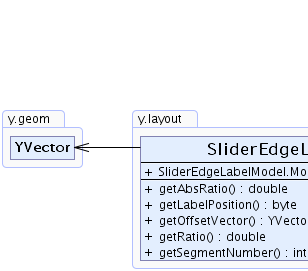Search this API

## y.layout Class SliderEdgeLabelModel.ModelParameter

```java.lang.Objecty.layout.SliderEdgeLabelModel.ModelParameter
```
Enclosing class:
SliderEdgeLabelModel

`public static class SliderEdgeLabelModel.ModelParameterextends java.lang.Object`

Encodes the model parameters for the two slider modes. The valid position are either `on the edge` or `beside the edge`.

The location of the label is described by the according segment index, the ratio of the position on the segment, the ratio of the position on the edge's path, an offset to the edge segment and the side of the edge.

`SliderEdgeLabelModel.getMode()`Constructor Summary
```SliderEdgeLabelModel.ModelParameter(int segmentNumber, double ratio, YVector offset, byte position, double absRatio)```
Creates a new instance of `SliderEdgeLabelModel.ModelParameter` using the given information.

Method Summary
` double` `getAbsRatio()`
Returns the label's absolute ratio with respect to the entire length of the edge path.
` byte` `getLabelPosition()`
Returns the label's position specifier that determines the side of the edge where the label is placed.
` YVector` `getOffsetVector()`
Returns the offset vector between the label and the edge segment relative to which it is placed.
` double` `getRatio()`
Returns the label's ratio with respect to the length of the edge segment relative to which it is placed.
` int` `getSegmentNumber()`
Returns the index of the edge segment relative to which the label is placed.

Methods inherited from class java.lang.Object
`clone, equals, finalize, getClass, hashCode, notify, notifyAll, toString, wait, wait, wait`

Constructor Detail

### SliderEdgeLabelModel.ModelParameter

```public SliderEdgeLabelModel.ModelParameter(int segmentNumber,
double ratio,
YVector offset,
byte position,
double absRatio)```
Creates a new instance of `SliderEdgeLabelModel.ModelParameter` using the given information.

Parameters:
`segmentNumber` - the zero-based index of the edge segment where the label should be placed
`ratio` - the ratio that describes the location relative to the segment where the label should be placed
`offset` - the offset vector between the label and the edge segment where it should be placed
`position` - one of the valid position specifiers
`absRatio` - the ratio that describes the location relative to the entire length of the edge where the label should be placed
Method Detail

### getSegmentNumber

`public int getSegmentNumber()`
Returns the index of the edge segment relative to which the label is placed.

The index is zero-based and starts at the edge's source end. Let `s` denote the number of edge segments. Then, a negative index value `i` denotes the segment with index `s + i`.

Returns:
the zero-based index of the edge segment where the label should be placed

### getRatio

`public double getRatio()`
Returns the label's ratio with respect to the length of the edge segment relative to which it is placed.

Returns:
the ratio that describes the location relative to the segment where the label should be placed

### getOffsetVector

`public YVector getOffsetVector()`
Returns the offset vector between the label and the edge segment relative to which it is placed. The offset vector describes the distance between the edge segment and the label's upper-left corner.

Returns:
the offset vector between the label and the edge segment where it should be placed

### getLabelPosition

`public byte getLabelPosition()`
Returns the label's position specifier that determines the side of the edge where the label is placed.

Returns:
one of the valid ratio specifiers

### getAbsRatio

`public double getAbsRatio()`
Returns the label's absolute ratio with respect to the entire length of the edge path.

Returns:
the ratio that describes the location relative to the entire length of the edge where the label should be placed
`getRatio()`